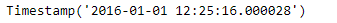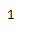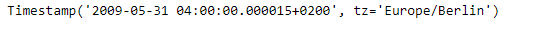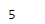# Python | Pandas Timestamp.month

• Last Updated : 08 Jan, 2019

Python is a great language for doing data analysis, primarily because of the fantastic ecosystem of data-centric python packages. Pandas is one of those packages and makes importing and analyzing data much easier.

Pandas` Timestamp.month` attribute is used to find the month value in the given Timestamp object.

Syntax : Timestamp.month

Parameters : None

Return : month

Example #1: Use `Timestamp.month` attribute to find the month value in the given Timestamp object.

 `# importing pandas as pd``import` `pandas as pd`` ` `# Create the Timestamp object``ts ``=` `pd.Timestamp(``2016``, ``1``, ``1``, ``12``, ``25``, ``16``, ``28``)`` ` `# Print the Timestamp object``print``(ts)`

Output :Now we will use the `Timestamp.month` attribute to print the value of month in ts object.

 `# find the value of month ``ts.month`

Output :As we can see in the output, the `Timestamp.month` attribute has returned 1 indicating that the value of month in the ts object is set to 1.

Example #2: Use `Timestamp.month` attribute to find the month value in the given Timestamp object.

 `# importing pandas as pd``import` `pandas as pd`` ` `# Create the Timestamp object``ts ``=` `pd.Timestamp(year ``=` `2009``, month ``=` `5``, day ``=` `31``, hour ``=` `4``, ``                      ``microsecond ``=` `15``, tz ``=` `'Europe/Berlin'``)`` ` `# Print the Timestamp object``print``(ts)`

Output :Now we will use the `Timestamp.month` attribute to print the value of month in ts object.

 `# find the value of month ``ts.month`

Output :As we can see in the output, the `Timestamp.month` attribute has returned 5 indicating that the value of month in the ts object is set to 5.

My Personal Notes arrow_drop_up